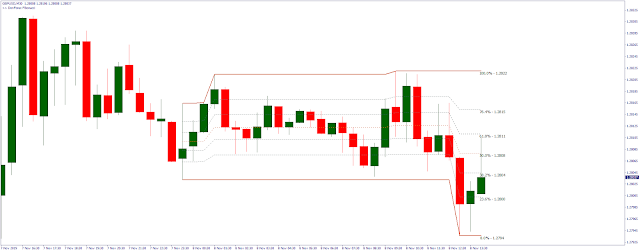# Don Forex Fibo (Fibonacci Indicator)

Don forex Fibo (Fibonacci indicator) is indicator that draw intraday (and intra-anytimeframe) Fibonacci-retracement tool. Adjustable base time frame.
How to use this tool?
Popular idea, that correction of the trend in a significant number of cases stops at Fibonacci numbers. This Fibo effect is often used in the prediction of the market. In the course of correction of trend movement, the
market often either rebounds, or completely unfolds at Fibonacci levels of 23.6, 38.2, 50, 61.8. With regard to daily trading, indicator use the Fibonacci grid, based on the latest 200 bars (you can change
it in the inputs). Typically, the price bounces off the levels of Fibo. The Fibonacci grid helps you better set Stop Loss and Take Profit levels. If you consider these levels as possible trend reversal/rebounds - you can
set Stop Loss a bit above the line and Take Profit a bit close a line.
Install Don Forex Fibo (Fibonacci indicator) instructions:
1. Unzip the *.zip file
2. Copy the indicator (*.ex4 file) into the {MT4 Data Folder}\MQL4\Indicators folder
3. Refresh the Navigator window (right click and Refresh) or restart the MT4
4. Open a chart and load the indicator